Share

# If you focus the image of a distant object, whose shape is given below, on a screen using a convex lens, the shape of the image of this object on the screen would be - CBSE Class 10 - Science

ConceptRefraction of Light Image Formation by Lenses - Convex Lens

#### Question

If you focus the image of a distant object, whose shape is given below, on a screen using a convex lens, the shape of the image of this object on the screen would be: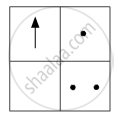(a)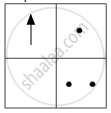(b)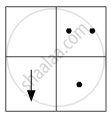(c)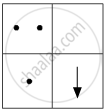(d)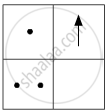#### Solution

As the image is focused on the screen thus the image formed is real. And, we know that real images are always inverted.

Thus, the shape of the image of the given pattern is represented by figure (b).

Hence, the correct answer isIs there an error in this question or solution?

#### Video TutorialsVIEW ALL 

Solution If you focus the image of a distant object, whose shape is given below, on a screen using a convex lens, the shape of the image of this object on the screen would be Concept: Refraction of Light - Image Formation by Lenses - Convex Lens.
S